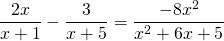Chapter 8: Rational Expressions

# 8.7 Solving Rational Equations

When solving equations that are made up of rational expressions, we use the same strategy we used to solve complex fractions, where the easiest solution involved multiplying by the LCD to remove the fractions. Consider the following examples.

Example 8.7.1

Solve the following: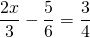For these three fractions, the LCD is 12. Therefore, multiply all parts of the equation by 12: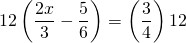This reduces the rational equation to: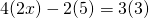Multiplying this out yields: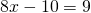Now isolate and solve for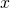: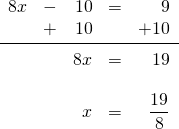Example 8.7.2

Solve the following: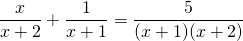For these three fractions, the LCD is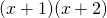. Therefore,  multiply all parts of the equation by: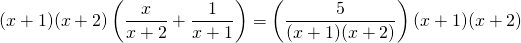This reduces the rational equation to: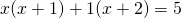Multiplying this out yields: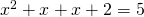Which reduces to: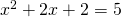Now subtract 5 from both sides of the equation to turn this into an equation that can be easily factored: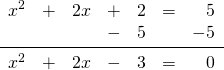This equation factors to: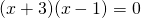The solutions are: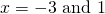# Questions

Solve each rational equation.

1.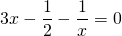2.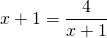3.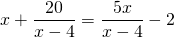4.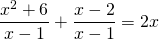5.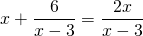6.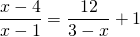7.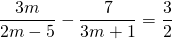8.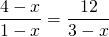9.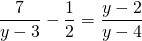10.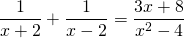11.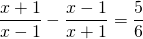12.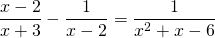13.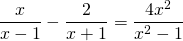14.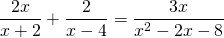15.